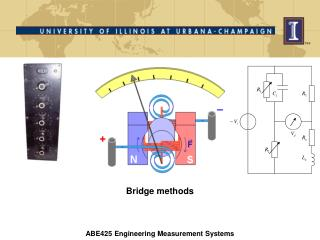Download PresentationBridge methods

# Bridge methods - PowerPoint PPT Presentation

Bridge methods. ABE425 Engineering Measurement Systems. Agenda. Null methods (calibration and highly accurate measurements) DC bridges (Resistance measurement) Wheatstone Capacitor and inductance (coil) models AC bridges (Inductance / capacitance measurement)I am the owner, or an agent authorized to act on behalf of the owner, of the copyrighted work described.
Download Presentation## Bridge methods

Download Policy: Content on the Website is provided to you AS IS for your information and personal use and may not be sold / licensed / shared on other websites without getting consent from its author.While downloading, if for some reason you are not able to download a presentation, the publisher may have deleted the file from their server.

- - - - - - - - - - - - - - - - - - - - - - - - - - E N D - - - - - - - - - - - - - - - - - - - - - - - - - -
Presentation Transcript
1. Bridge methods ABE425 Engineering Measurement Systems

2. Agenda Null methods (calibration and highly accurate measurements) DC bridges (Resistance measurement) Wheatstone Capacitor and inductance (coil) models AC bridges (Inductance / capacitance measurement) Null-type Parallel-Resistance-Capacitance bridge for capacitance and dissipation factor measurement Maxwell bridge for inductance (coil) and quality factor measurement Wien bridge Approximate measurement of Inductance and Capacitance Deflection methods (control systems) Deflection-type Wheatstone bridge and non-linearity

3. Null-type DC Wheatstone bridges are used for accurate resistance measurement The bridge is balanced when the voltage Vdis adjusted to zero by tuning R1 while R2 and R3 are known and kept constant. The null-detector is usually some type of galvanometer The unknown resistance value can then be computed using the values of the other resistances Since there are no inductances (coils) or capacitances, a DC source is sufficient This type of bridge is used for strain gage measurements

4. Measurement procedure using Galvanometer and decade resistor box Decade Box Galvanometer Known, constant At balance: Unknown resistor

5. Galvanometers are VERY SENSITIVE instruments to detect zero current D'Arsonval galvanometer

6. Thompson mirror type galvanometer (1880). Note the ‘antenna’ to compensate for electric/magnetic fields

7. Null-type AC Wheatstone bridge for impedance measurement The bridge is balanced when the voltage Vdis adjusted to zero by tuning

8. Capacitor and Inductance (coil) models Capacitor model with Capacitance and dissipation resistance Inductor (coil) model with Inductance and series resistance Inductances have a quality factor

9. Null-type Parallel-Resistance-Capacitance bridge for capacitance and dissipation factor measurement

10. Maxwell bridge to measure inductance, resistance and quality factor of low quality coils (Q<10)

11. Hay bridge to measure inductance, resistance and quality factor of high quality coils (Q>10)

12. Wien bridge for frequency measurement

13. The coil characteristics inductance and series resistance can be measured by equalizing the voltage across a variable resistor and the coil itself Series resistance of the coil RL measured with a DVM

14. Approximate method of measuring capacitance Measure the AC Voltages for a known input frequency across resistor R and capacitor C Resistance measured with a DVM

15. Deflection type DC Wheatstone bridge

16. Output (deflection) for R2, R3 = 1,000 Ohm showing significant non-linearity Bridge balance

17. Output (deflection) for R2, R3 = 10,000 Ohm showing reduced non-linearity Bridge balance

18. Measuring the drag coefficient of a sphere using a compensation method Electric current returns sphere to original position Air flow pushes sphere to the right Drag coefficient ~ Electric current

19. Links Schneider, N. 1904. Electrical instruments and testing. Spon and Chamberlain, New York.

20. The End